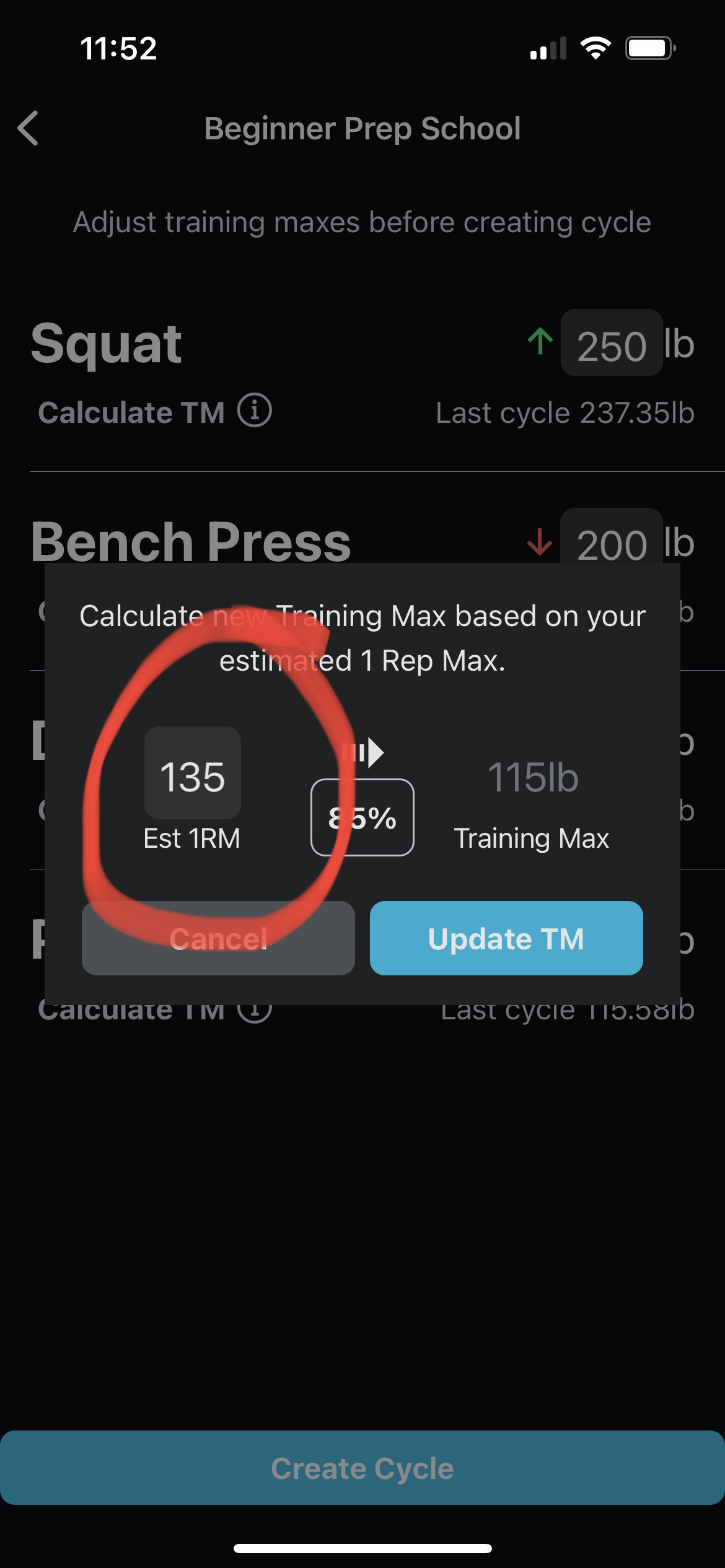# How do I calculate 1 Rep Max using a formula?

When creating a new cycle, tap "Calculate TM" below the exercise name. A pop-up will display showing a prefilled 1 Rep Max (1RM). The prefilled 1RM number is calculated using the following formula:

1RM = (Weight) * (Reps) * (0.0333) + (Weight)